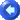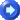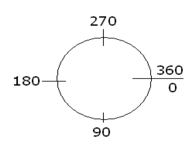Angles and DegreesAngles are measured in degrees.  An angle of 0 is in the direction of the positive X-axis.  An angle of 90 degrees is in the direction of the positive Y-axis.  Angles increase in the direction from the positive X-axis toward the positive Y-axis.  This means angles increase in a clockwise direction.When an object asks for an angle "sweep," like that of an arc or a pie wedge, this is referring to how big you want the angle to be.  For example, if you wanted an arc to be 1/4 of the way around the ellipse, you would enter in a sweep of 90 degrees.  If you wanted it to be 3/4 of the way around, you would enter in a sweep of 270 because from 0 around to 270 is 3/4 of the circle.  For an angle sweep, the degree location on a circle is irrelevant.  You would use degree location like the one above for where you would want a wedge or arc to start.  You would enter the degrees for its starting point next to "Wedge Angle" or "Arc Angle."

Pie Wedges

Arcs